2D plots
3D plots
Multilayer Plots

# 2D plots

A 2D plot is based on curves which are defined by Y values as functions of X values. There are two ways to obtain a 2D plot depending on the way the (X,Y) values are defined:

• You can have your (X,Y) values in a table. You need to select at least one column as X values and one column as Y values. This is specified using the "Plot Designation" option found in the Column Options... command. Then you select the columns and use one of the commands in the Plot menu to plot the data.

• If you want to plot a function, you don't need a table at all. You can plot the function directly with the New Function Plot... command. This opens the corresponding dialog box where you define the mathematical expression of your function.

• These two methods can be combined by first defining a table, and then filling it with the results of evaluating your function. This is done with the Set Column Values... command. Then you select the columns and use one of the commands from the Plot menu to plot the data.

In each of these cases, QtiPlot will create a new graph with the plotted curve placed on a new layer. Data plots and function plots can also be added to an existing layer using either the New Function Plot... command command or by right clicking within the area of the desired plot to pop up the plot's Graph Menu, and then selecting Add...Add Function.

Once the plot is created, you can customize all the graphic items in the plot using commands from the Format Menu. You can add new items (text labels, lines or arrows, new legend, images) to the plot with the commands of the Graph Menu.

## 2D plot from data.

The data must be stored in a table. There are two methods for inserting your (X,Y) values into the table: you can type them directly from the keyboard, or you can read them from a file. Here we will use the first solution, refer to the Import -> Import ASCII... command to use the second.

The first step in this example is to create an empty project with the New Project command from the File menu. You can also use the Ctrl-N key or the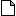icon from the File toolbar. Next create a new table using the New Table command from the File menu, the Ctrl-T key, or the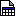icon from the File toolbar.

A newly created table has two columns (one for X and one for Y) and 30 rows. You can add rows and columns by selecting a row or a column and using the right mouse button. You can also modify the number of rows and columns with the Rows and Columns from the Table menu. Try setting the number of rows to 7, which will match the table shown below. Then enter the values as shown (you can of course use your own data). You should now have this table:

Figure 2-1. A simple 2D plot: the table.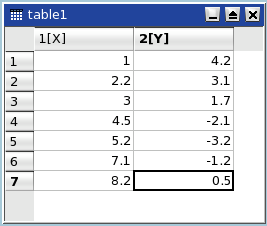You must next select the data to be plotted. To select the 2 columns of data just entered, left click on the title of first column and drag the mouse pointer over to the title of the second column while holding the left mouse button down. Now, with the columns selected, you can build the plot (here a simple 2D scatter) with the Scatter command from the context menu, or by clicking on the correspondingicon from the Plot toolbar or with the Scatter command from the Plot menu. A plot is created in the plotting area of a new layer on a new graph. Default options are used for for all newly created elements. You can customize the default options with the preferences dialog. The default options will produce the following:

Figure 2-2. A simple 2D plot: the default plot.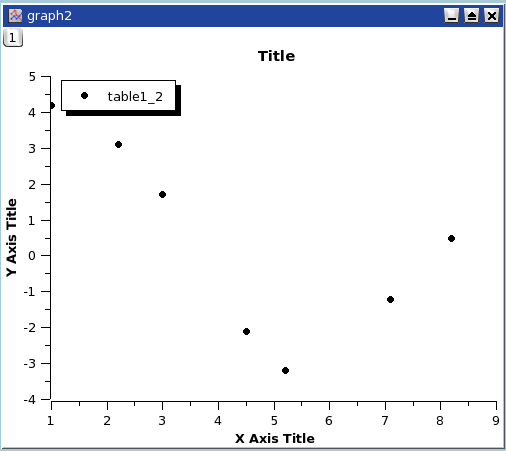You can now customize your plot and the elements of the parent layer. Double clicking on any point will open the Custom curves dialog, which is used to modify the plotted symbols. A double-click on any axis opens the general plot options dialog, where you can change scales, fonts for the axis labels, etc. You can also add grid lines on X or Y axes, etc. Finally, a double click on any text item (X title, Y title, plot title) allows you to change the text and its presentation. As an illustration, several changes have been made to the above plot. The final result is:

Figure 2-3. A simple 2D plot: the plot finished.Finally, you should save your project in a '.qti' file using the Save Project command from the File menu or by typing the Ctrl-S key, or by clicking the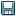icon from the File toolbar. Depending on your needs, you can export the plot in any of several standard image file formats using the Export Graph -> Current command from the File menu, or by entering the Alt-G key.

There are several types of curves which can be plotted from a table. They are presented in the Plot menu

It is possible to use up to four axes for the data:

Figure 2-4. A 2D plot with two Y axes.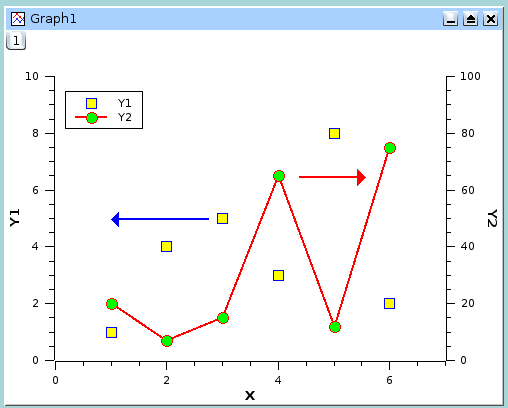In addition to the customizations which have been already been described, for the figure above the axes used for each curve were defined using the Custom Curves Dialog, and two arrows were added with the Draw Arrow. Note that the table must be modified by the addition of a second column of Y data before the second curve can be drawn in the plotting area (using Graph Menu, and then selecting Add...Add/Remove Curve).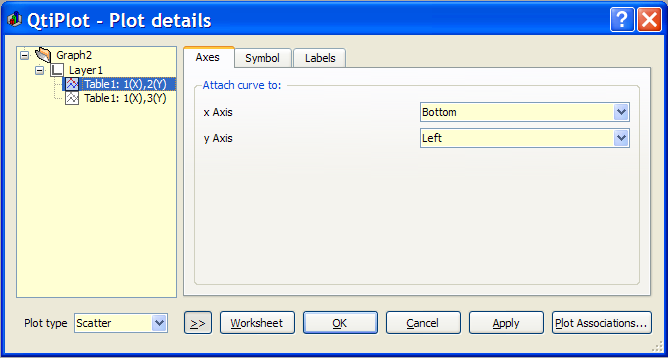## 2D plot from function.

There are two ways to obtain such a plot: you can plot a function directly, or fill a table with the values calculated from a function and create the plot in the usual way.

### Direct plot of a function.

If you just want to plot a function, you can use the New Function Plot... command from the File menu, click the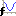icon in the File toolbar, or simply enter Ctrl-F.

This command will open the Add Function Curve dialog. You can then enter the mathematical expression of your function, the X range to be used for the plot, and the number of points in the X range. Besides classical Y=f(X) functions, you can also define parametric and polar functions.

Figure 2-5. Direct plot of a function.### Filling of a table with the values of a function.

If you want to work not only with the plot but also with the resulting data, create a new table as explained in the previous section. Then fill this table with the values of the function evaluation using the Set Column Values... command.

Let's obtain the same plot as in the previous example. Create a new table (key Ctrl-T), select the first column and use the Set Column Values... command either from the context menu, or the Table menu. The row number can be used in functions by referencing the row number symbol, i. For a range of 0.01-30 in 300 steps (0.01 per step) enter the function expression i/10 and use 300 rows. (Note that since row numbering starts at 1, to actually get the X range used in the last example (0-30 over 300 points), we would need to define the function expression as (i-1)*30/299.)

Figure 2-6. Function plot: filling of the X column.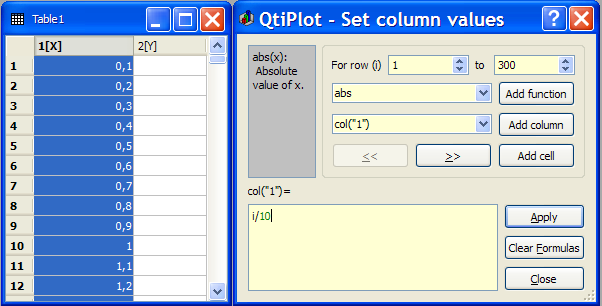The second step is to select the second (Y) column and use the Set Column Values... command to set up the function. The expression is a function of the X values (that is the first column) which is named col(1). Enter sin(col("1"))+cos(col("1")/3+1) as the function and click apply to generate the values in the Y column.

Figure 2-7. Function plot: filling of the Y column.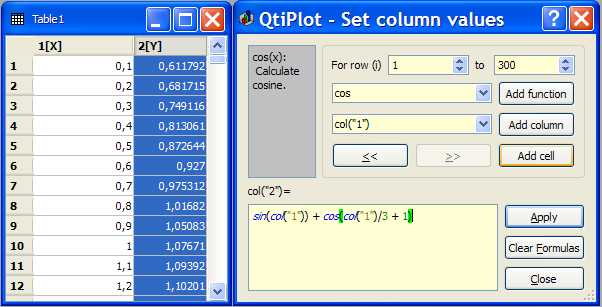Once the table is ready, you just have to build the plot as explained in the previous section.